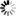Welcome to Central Library, SUST
Normal view

# Calculus for Computer Graphics [electronic resource] / by John Vince.

Material type:TextPublisher: London : Springer London : Imprint: Springer, 2013Description: XIII, 227 p. 144 illus. online resourceContent type:
• text
Media type:
• computer
Carrier type:
• online resource
ISBN:
• 9781447154662
Additional physical formats: Printed edition:: No titleDDC classification:
• 006.6 23
LOC classification:
• T385
Online resources:
Contents:
Preface -- Introduction -- Functions -- Limits and Derivatives -- Derivatives and Antiderivatives -- Higher Derivatives -- Partial Derivatives -- Integral Calculus -- Area Under a Graph -- Arc Length -- Surface Area -- Volume -- Vector-Valued Functions -- Conclusion -- Appendices -- Index.
Summary: Students studying computer animation and computer games have to be familiar with geometry, matrices, vectors, rotation transforms, quaternions, curves and surfaces, and as computer graphics software becomes increasingly sophisticated, calculus is also being used to resolve its associated problems. The author draws upon his experience in teaching mathematics to undergraduates to make calculus appear no more challenging than any other branch of mathematics. He introduces the subject by examining how functions depend upon their independent variables, and then derives the appropriate mathematical underpinning and definitions. This gives rise to a function’s derivative and its antiderivative, or integral. Using the idea of limits, the reader is introduced to derivatives and integrals of many common functions. Other chapters address higher-order derivatives, partial derivatives, Jacobians, vector-based functions, single, double and triple integrals, with numerous worked examples, and over a hundred illustrations. Calculus for Computer Graphics complements the author’s other books on mathematics for computer graphics, and assumes that the reader is familiar with everyday algebra, trigonometry, vectors and determinants. After studying this book, the reader should understand calculus and its application within the world of computer games and animation.
Tags from this library: No tags from this library for this title.
Star ratingsAverage rating: 0.0 (0 votes)
No physical items for this record

Preface -- Introduction -- Functions -- Limits and Derivatives -- Derivatives and Antiderivatives -- Higher Derivatives -- Partial Derivatives -- Integral Calculus -- Area Under a Graph -- Arc Length -- Surface Area -- Volume -- Vector-Valued Functions -- Conclusion -- Appendices -- Index.

Students studying computer animation and computer games have to be familiar with geometry, matrices, vectors, rotation transforms, quaternions, curves and surfaces, and as computer graphics software becomes increasingly sophisticated, calculus is also being used to resolve its associated problems. The author draws upon his experience in teaching mathematics to undergraduates to make calculus appear no more challenging than any other branch of mathematics. He introduces the subject by examining how functions depend upon their independent variables, and then derives the appropriate mathematical underpinning and definitions. This gives rise to a function’s derivative and its antiderivative, or integral. Using the idea of limits, the reader is introduced to derivatives and integrals of many common functions. Other chapters address higher-order derivatives, partial derivatives, Jacobians, vector-based functions, single, double and triple integrals, with numerous worked examples, and over a hundred illustrations. Calculus for Computer Graphics complements the author’s other books on mathematics for computer graphics, and assumes that the reader is familiar with everyday algebra, trigonometry, vectors and determinants. After studying this book, the reader should understand calculus and its application within the world of computer games and animation.

There are no comments on this title.